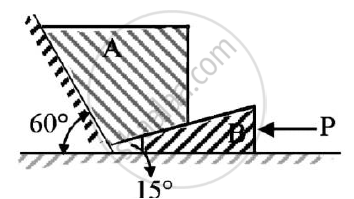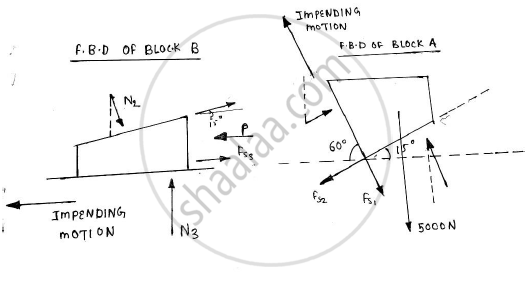# Determine the Force P Required to Move the Block a of 5000 N Weight up the Inclined Plane, Coefficient of Friction Between All Contact Surfaces is 0.25. Neglect the Weight of the Wedge and the - Engineering Mechanics

Determine the force P required to move the block A of 5000 N weight up the inclined plane, coefficient of friction between all contact surfaces is 0.25. Neglect the weight of the wedge and the wedge angle is 15 degrees.Given : Weight of block A = 5000 N
μs=0.25
Wedge angle = 15º

To find : Force P required to move block A up the inclined plane#### Solution

The impending motion of block A is to move up
The block A is in equilibrium
N1,N2,N3 are the normal reactions
Fs1 = μ1N1 = 0.25N1
Fs2 = μ2N2 = 0.25N2
Fs3 = μ3N3 = 0.25N3
Applying the conditions of equilibrium
ΣFy = 0
∴ -5000 + N1 cos 60 – Fs1 sin 60 – Fs2 sin 15 + N2 cos 15 = 0
∴ N1 x 0.5 – 0.25N1 x 0.866 -0.25 N2 x 0.2588 + N2 x 0.9659 = 5000 (From 1)
∴ 0.2835 N1 +0.9012 N2 = 5000 ……………..(2)
Applying the conditions of equilibrium
ΣFx = 0
∴N1 sin 60 +Fs1 cos 60 –Fs2 cos 15 – N2 sin 15 =0
∴ 0.866 N1 + 0.25 x N1 x 0.5 -0.25 x N2 x 0.9659 –N2 x 0.2588 = 0(From 1)
∴ 0.991 N1 -0.5003 N2 = 0
Solving equation, no 2 and 3
N1 = 2417.0851 N
N2 = 4787.79 N
The impending motion of block B is towards left Block B is in equilibrium. Applying the conditions of equilibrium
ΣFy = 0
∴ N3 + Fs2 sin 15 –N2 cos 15 = 0
∴N3 + 0.25N2 x 0.2588 – N2 x 0.9659 = 0
∴ N3 – 0.9012 N2 = 0
∴ N3= 0.9012 x 4787.79 = 4314.7563
Applying conditions of equilibrium
ΣFx = 0
∴ -P + Fs3 +Fs2 cos 15 +N2 sin 5 =0
∴ 0.25 N3 +0.25 N2 x 0.9659 +N2 x 0.2588 =P
∴ P = 0.25 N3 +0.5003 N2 = 0.25 X 4314.7563 + 0.5003 x 4787.79 = 3474 N

The force P required to move the block A of weight 5000 N up the inclined plane is P=3474 N

Concept: Condition of equilibrium for concurrent forces
Is there an error in this question or solution?
2017-2018 (December) CBCGS

Share